Reach Us+44-1522-440391
Mixtures of Self-Modelling Regressions | OMICS International
Journal of Biometrics & Biostatistics

Like us on:

All submissions will be redirected to Online Manuscript Submission System. Authors are requested to submit articles directly to Online Manuscript Submission System of respective journal.

# Mixtures of Self-Modelling Regressions

Rhonda D Szczesniak1*, Kert Viele2,3 and Robin L Cooper4

1Division of Biostatistics and Epidemiology, Cincinnati Children's Hospital Medical Center, USA

2Berry Consultants, LLC, USA

3Department of Statistics, University of Kentucky, USA

4Department of Biology, University of Kentucky, USA

*Corresponding Author:
Rhonda D Szczesniak
Division of Biostatistics & Epidemiology
Cincinnati Children's Hospital Medical Center, USA
Tel: 513-803-0563
E-mail: [email protected]

Received date: July 02, 2014; Accepted date: August 08, 2014; Published date: August 15, 2014

Citation: Szczesniak RD, Viele K, Cooper RL (2014) Mixtures of Self-Modelling Regressions. J Biom Biostat S12:003. doi:10.4172/2155-6180.S12-003

Copyright: © 2014 Szczesniak RD, et al. This is an open-access article distributed under the terms of the Creative Commons Attribution License, which permits unrestricted use, distribution, and reproduction in any medium, provided the original author and source are are credited.

Visit for more related articles at Journal of Biometrics & Biostatistics

#### Abstract

A shape invariant model for functions f1,…,fn specifies that each individual function fi can be related to a common shape function g through the relation fi(x)=aig(cix + di) + bi. We consider a flexible mixture model that allows multiple shape functions g1,…,gK, where each fi is a shape invariant transformation of one of those gk. We derive an MCMC algorithm for fitting the model using Bayesian Adaptive Regression Splines (BARS), propose a strategy to improve its mixing properties and utilize existing model selection criteria in semiparametric mixtures to select the number of distinct shape functions. We discuss some of the computational difficulties that arise. The method is illustrated using synaptic transmission data, where the groups of functions may indicate different active zones in a synapse.

#### Introduction

Self-Modeling regressions form a class of models [1,2] for functional data observed for many individuals. We observe data, where the subject specific functions fi(x) (sometimes referred to as traces) have the form where g is a base function and θi is a subject specific parameter which specifies a transformation connecting g and the functions fi. A variety of choices is available for this transformation class which unites the subject specific functions fi to g. One of the first examples in the literature, which we will address here, is the shape invariant model, referred to as SIM (1), where θi=(ai,bi,ci,di) are called self-modeling coefficients and

fi(x)=aig(cix + di) + bi

The self-modeling coefficients result in fi being an affine transformation of g in the x and y axes. Other types of transformations have been shown which use a Bayesian warping function method . Altman and Villarreal  have developed the SIM where interest centers on variation in the self-modeling coefficients, using nonlinear mixed effects  and p-splines to model the θi parameters. More recent work involved inferential comparisons on θi in the SIM setting . In this work, our primary objectives are the following:

1. To consider mixtures of shape invariant models (MSIMs) where there is more than one underlying shape function g. Unknown to the investigator, suppose the subject specific functions may be grouped so that one group is defined by shape invariant transformations of an underlying g1, another group is defined by shape invariant transformation of an underlying g2, and so on. A motivating example follows below.

2. To utilize Bayesian Adaptive Regression Splines (BARS) [7-9] to estimate the underlying shape functions gk for k=1,…,K. BARS has been shown to provide parsimonious fits (e.g. fewer knots) of complicated functions. Several computational challenges arise in these endeavors which are described in Implementation.

In our motivating example, data are obtained from a recording of synaptic transmission at a neuromuscular junction (NMJ) from a crayfish (Figure 1a). The motor nerve is repeatedly stimulated and the synaptic response is monitored as described previously in work by two of the authors . With low frequency stimulation of this low output NMJ, occasionally an evoked response is observed. In the sample shown, 61 events occurred in 1000 stimulations. After some data cleaning  our data of interest is the 61 functions describing voltage over a dense series of time points.

Figure 1: A set of 61 evoked excitatory post-synaptic potentials (EPSPs) Data in the left pane (a) describe the evoked voltage responses observed at the crayfish neuromuscular junction from 1000 stimulation trials. While the functions vary in height, width, and peak locations, all the functions have a similar underlying shape. The right pane (b) shows aligned firings with the estimated underlying shape g in red. These are the same data from (a) aligned to each other.

These functions vary in terms of their heights, widths, and peak locations, but in fact do all have similar shapes as shown by the aligned curves which result from fitting a SIM model to all the functions (Figure 1b). The details of this fit, which simply assumed known knots for a spline fit to g, are described in previous work . This fit accounts for over 96% of the variation in the dataset.

Biologically, the variation between the functions is assumed to occur because of aspects related to the physiology and structure of the synapses at the NMJ. The electrical signal in the neuron is translated into a vesicle fusing with the presynaptic membrane at active zones to release neurotransmitters. The postsynaptic receptors on the muscle detect the transmitter which results in a depolarization of the muscle fiber. There are various possibilities for the variation in quantal responses. Some of those possibilities involve the location where neurotransmitter is released and such variation would result in two or more groups of functions appearing in the data. Thus, finding groups of functions in the data may indicate multiple sites active in the synapse.

In searching for different sites, we wish to find groups of functions within the 61 functions shown in Figure 1a. This is our motivation for fitting a mixture of shape invariant functions, where the different underlying shape functions may indicate different active zones. Previous work in this area has uncovered groupings based on functionals of the traces, for example by fitting a normal mixture model on the peak amplitudes . Of course, individual functionals only utilize a small amount of the information available in the entire voltage function. It would also be possible to fit a single shape function g and then fit a mixture model over the parameters (a,b,c,d), which we do not pursue here.

A brief outline of this paper is as follows. In the next section we introduce MSIMs. We describe our computational techniques, including necessary adjustments to the BARS algorithm, in Implementation. In we then describe methods for evaluating the results (this is nontrivial due to identifiability issues present in SIMs in general), increasing MCMC mixing and selecting the number of components in the mixture. In Results we discuss the results of the simulations and the analysis of the data in Figure 1a. Finally, we provide a discussion of the results.

#### Mixtures of Shape Invariant Models

Prior and likelihood structure

Suppose we observe I individuals and for each individual we observe data pairs (xij,yij) for i =1,…,I and j=1,…,Ji. We also assume there exist underlying shape functions g1,…,gK such that the ith individual follows shape gk with probability pk, where p=(p1,…,pK) is a vector of probabilities. Let zi be a group indicator where zi=k indicates that the ith individual follows the kth function and that θi=(ai,bi,ci,di) are the self-modeling coefficients for the ith individual. Finally σ2 is the error variance. Where possible, we place conditionally conjugate priors on the parameters to ensure an easier MCMC implementation. In what follows g refers to the collection of g1,…,gK and θ refers to the collection of θ1,…, θI, and N(μ,σ2)(x) refers to a normal density with mean μ and variance σ2 evaluated at x, with similar notation for other densities. The general prior and likelihood structure is below. We will describe the priors on the θi and gk separately.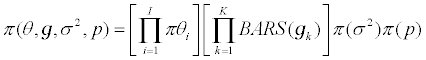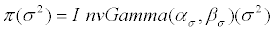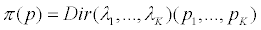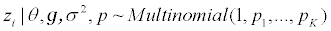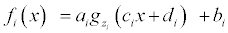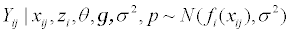Our inferential goals are to estimate the underlying shape functions, g1,…,gK, the self-modeling coefficients (ai,bi,ci,di)=θi for each individual function fi, the posterior probabilities of each individual function belonging to each group, and common error variance σ2.

Priors on shape functions, gk

We utilize BARS to estimate each of the gk functions. Each gk has a B-spline basis expansion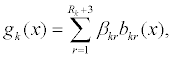where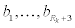form a B-spline basis with knots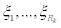and spline coefficient vector β. BARS utilizes a reversible jump MCMC (commonly referred to as RJMCMC) algorithm which samples over the knots in a B-spline used to estimate the function of interest. Without loss of generality, assume the function of interest (for us one of the gk functions) is supported over the unit interval (0,1). A B-spline basis is formed using a vector of knots. The prior on this vector assumes the number of knots follows a distribution and that the individual knots are uniformly distributed throughout (0,1). BARS proceeds by iteratively adding, removing, and relocating knots. These changes in the knot structure are accepted or rejected according to BIC (BIC uses the maximum likelihood estimates of the spline coefficients). As BIC is used, this is very close asymptotically to using a unit-information prior  on the spline coefficients β. This structure is what is intended by the notation BARS(gk) used above.

Priors on the self-modeling coefficients

Finally, for estimating the self-modeling coefficients θi = (ai,bi,ci,di), we place a vague bivariate normal prior on ai and bi (these parameters act as regression coefficients). In general, there is no conditionally conjugate prior for ci and di. For functions with a single dominant peak, a transformation of ci and di to a location-scale family is useful.

Suppose for example the underlying shape function g has a peak at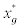. The individual function fi would have that peak at the x where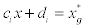which is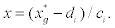Note that changes in either ci or di result in changes to the peak location of fi. As proper alignment of the peak is very important to achieving a high likelihood, this induces a correlation in the posterior distribution of ci and di. We have found better results (in terms of the mixing properties of the MCMC chain) by transforming to ci (which controls the "spread" of the curve) and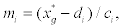which represents the location of the peak. Thus, instead of fi(x) = aig(cix + di) + bi, we use the transformation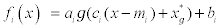. For a more thorough description of curve registration techniques and other, potentially helpful transformations for improving MCMC mixing, we refer the reader to the original paper by Ramsay and Li  and Gilks and Roberts , respectively.

To see the benefit of this transformation by example, let g be a triangular "tent function" where g(x)=0 outside (0.2,0.6), g(x) increases linearly from 0 to 1 over (0.2,0.4) and decreases linearly from 1 to 0 over (0.4,0.6). We generated data using f(x)=g(0.5x−0.25) for x=(0, 0.01,0.02,…,2) and N(0,1) error. The peak of g occurs at 0.4 and the corresponding peak of f occurs at m=1.3. Keeping everything fixed at the true value except c and d, Figure 2a shows the loglikelihood over (c,d). As can be seen, there is a distinct correlation between c and d which limits the mixing of the MCMC chain. In contrast, Figure 2b shows the loglikelihood as a function of c and m, which provides a much better structure for MCMC.

Figure 2: Loglikelihood structures of shift-scale and location-scale selfmodeling coefficients. The left pane (a) shows the structure for the (c,d) selfmodeling coefficients, compared to the right pane(b) which shows the (c,m) location-scale transformation. For a function with a single dominant peak, the location-scale transformation has a much lower correlation between c and m and thus the correspondingMCMC algorithm has superior mixing properties.

Of course, this transformation is limited to g with a single peak, which is not always true. Thus, this transformation will not produce an improvement in general, or other transformations may be useful in other contexts. For our synaptic transmission data, we have found this transformation to be quite useful. Thus, for data with a dominant peak: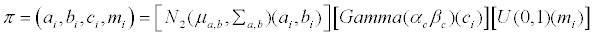Identifiability

Identifiability is a pervasive issue in self-modeling regressions [2,4]. Suppose for example we have a particular underlying shape function g(x) and a set of coefficients (a,b,c,d). One could achieve the same f by taking g`(x)=2g(x) and taking a`=a/2. Generally, one can take any self-modeling transformation of g and then adjust (a,b,c,d) appropriately to produce the same f. In the original Lawton paper (1), this problem was addressed by forcing g to have particular properties (such as a maximum of 1 and minimum of 0). However, in the Bayesian formulation such constraints would require any estimation method to be significantly altered. BARS, which we utilize here, is not obviously adjustable to handle constraints on the function.

An alternative method of forcing identifiability is to pick an individual function fi and force its corresponding (a,b,c,d) to be (1,0,1,0). This method is utilized in previous work . This forces the scaling on g to follow the chosen function but allows BARS to run unconstrained. The central theme here is that in the self-modeling paradigm you are really estimating relative shifts and scalings among the functions.

Unfortunately, in the mixture setup this is not feasible, as there are multiple shape functions. Since we do not know a priori which functions, f1,…,fI belong to which group (e.g. which are self-modeling versions of g1, which are self-modeling versions of g2, etc.) we cannot select functions to use as "anchors" in each group. Thus the simplest option seems to be to leave a nonidentified model. Altman and Villarreal  also use an unconstrained formulation without difficulty, though from a frequentist standpoint.

Note, however, that our central inferential goals are the assignment of functions to groups, the estimation of the individual functions, and the underlying shape of the g1,…,gK functions. The first two are identifiable, and g1,…,gK are identifiable up to self modeling versions. As with any mixture model, there is a secondary identifiability problem in that the component labels may be switched, but component label switching  has not been an issue in the analyses we performed. Although not addressed in this work, there is a third identifiability problem in mixture models arising from having an unnecessarily large number of mixtures when a smaller number of mixtures is actually sufficient.

Implementation

We implemented the model in Section 2 using an MCMC scheme. Some parameters are of course easier to update than others.

Sampling scheme

Updating σ2 and p: Both σ2 and p have straight forward conjugate priors conditional on the rest of the parameters.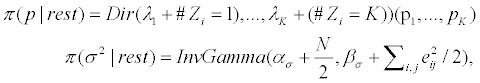(1)

whereShape functionsg1,…,gk: Conditional on the remaining variables, each gk is conditionally independent, with each gk being updated separately using only those data functions with zi=k.

Each gk is defined by its corresponding knot set ξk and spline coefficients βk. In the original BARS algorithm , updating was done entirely on ξ using BIC with the maximum likelihood estimate of β. While we update ξ using the BARS updating, we actually draw ξ,β| rest via ξ|rest and β|ξ, rest. Our reason for drawing β values is that, unlike the original work , we have additional parameters (specifically zi and θi) that are easier to simulate if β is fixed.

Updating Zi: Updating each Zi also requires moving functions between groups, as there are subject specific parameters θi for each variable. In the MCMC scheme one must take into account that (a,b,c,d) have different interpretations depending on which group the function belongs to (e.g. the outcome of Zi ). If g1 and g2 have different peaks, for example, then a perfect fit for g1 would require a much different value of a than a perfect fit for g2.

Ideally, we would like to avoid this problem and integrate the selfmodeling coefficients out to find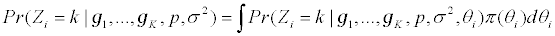Unfortunately, it is not computationally efficient to find this integral nor is it efficient to approximate it (for example finding the MLE of θi would require numerical maximization). Thus in our sampler we utilize θik (different θi for each k). To draw a new Zi, note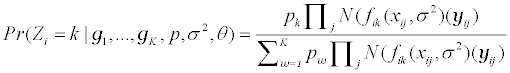where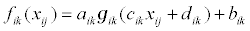Updating the self-modeling coefficients: Finally, the self-modeling coefficients (a,b) (recall in the sampler we have separate self-modeling coefficients θik for each i and k) are updated straightforwardly, as they are regression parameters with conjugate priors. The (c,m) coefficients are updated using a Metropolis-Hastings random walk scheme.

Singularities in the BARS updating

In the original BARS implementation, proposed knot sets which resulted in singular design matrices (loosely, too many knots in an area with too few data points), were discarded [16,17]; these proposals may be viewed simply as a data dependent prior. In the self-modeling setup, there is an additional complication. In one iteration we may achieve a nonsingular design matrix, but as the self-modeling coefficients θi are adjusted, this moves mi defined in Section 2.1.2 and may result in a singularity in the design matrix. We additionally reject these moves out of hand in the sampler. At present, these rejections in the sampler are not a frequent occurrence, but seems an unavoidable problem as long as BIC is used in the BARS steps.

Starting values

To initialize the chain, all fi functions were first aligned to the largest function (the trace with the largest difference between the maximum and minimum values) using a Procrustes registration . We then computed the mean square error (MSE) between each aligned trace and the largest trace. Focusing on a two-component mixture, the half of the traces with the smallest MSE values were placed in one group while the half of the traces with the largest MSE values were placed in the second group. Essentially, this means the half of the traces closest in shape (not necessarily scale) to the largest trace were placed together in a group. This initialized the Zi values. To initialize g1 and g2 in the two component mixture model, we fit a spline to the traces in each group (we simply assume 9 equally spaced knots initially, which is then adjusted by the sampler). The initial spline coefficients are then computed by aligning each individual trace to its corresponding gZiand finally the error variance is computed by taking the MSE of the entire fit.

#### Assessing Implementation Results

Posterior inference

At the end of the MCMC run we have a set of M iterations. For each m=1,…,M, the mth iteration contains functions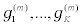(expressed in a spline basis), mixing proportions p(m), an error standard deviation σ(m) and component assignments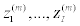for each individual function. Finally, for each i we have self-modeling coefficients θi(m). We assess the results in terms of the identifiable pieces of the sampler.

1. We directly have the mixing proportions p between the different functions gk. We also compute the estimated posterior probability each individual trace belongs to each group by finding the observed proportion of times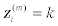for each k. Thus, we acquire overall proportions of functions in each group and the estimated posterior probabilities each individual function belongs to each individual gk.

2. To assess the fit of the individual functions, we computed the estimated fi(t) by constructing for each iteration and each trace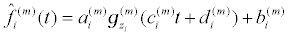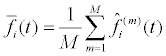(2)

In the plots that are constructed from the simulation studies and EPSPs application in Section 5, we plot a selection of the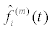functions (iterations m = 100,200,…) and the overall average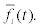To estimate each gk we must remember that each gk is allowed to drift in the sampler, and thus the resulting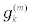iterations must be aligned in shape before producing an estimate. Thus, we begin by taking the functionsacross the iterations and aligning them to each other. This was done via a Procrustes registration as in previous work . The base shape function of this alignment was used as the estimate for gk.

The amount of burn in necessary for Gibbs sampling in the MSIM setting depends on data-specific and method-specific factors. Wallstrom et al.  recommend up to 20,000 burn-in iterations for the BARS implementation with normally-distributed data. We found that additional iterations were necessary to achieve proper burn in for the self-modeling coefficients.

Improving acceptance rates

It is important that the sampling mechanism provide a sufficient amount of switching in order to explore the state space well. Typically, one would choose a reversible-jump mechanism to facilitate this switching, but in most RJMCMC scenarios it is easier to sample from the posterior distribution within each subgroup (i.e. mixture component). The aforementioned sampling mechanism in Section 3 uses metropolis random walks within each subgroup, which may lead to lower acceptance rates, thereby lowering the degree of switching between groups.

To facilitate switching between groups, we propose using the approximating posterior distributions of the self-modeling coefficients in θi from our previous MCMC implementation in Section 3 to conduct a second MCMC. In this second MCMC, acceptance rates may be improved as follows. Let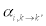be the probability of the ith trace moving from the current state Mk to the candidate state Mk’. For ease of description, we will now omit notation for trace i. The acceptance probability for moving from the current state (k) to the candidate state (k’) is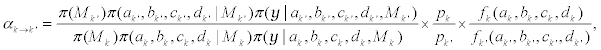(3)

where in general notation for state m,π (Mm) is the prior probability that the trace has underlying shape function gm; fm() refers to coefficients (am,bm,cm,dm) being evaluated at the multivariate normal distribution with respective mean vector pm and covariance matrix Σm obtained from the complete conditional distributions in the first MCMC implementation; pm is the empirical probability that the trace belonged with shape function gm() in the first MCMC; π(y|am,bm,cm,dm,Mm) is the likelihood for the trace under shape function gm. The π(am,bm,cm,dm|Mm) corresponds to coefficients (am,bm,cm,dm) being evaluated using priors under Mm, which can be simplified as π(am,bm,cm,dm|Mm)= π(am,bm,cm,dm|Mm)π(cm|Mm)π(dm|Mm); prior π(dm|Mm) is an induced prior that we compute via transformations. The algorithm for computing the acceptance probabilities is outlined in Supplementary Information.

Determining the number of mixtures

One outstanding issue still to be addressed is inferentially determining the number of groups present in the data. When making this determination, BIC is intuitively a reasonable choice; however, regularity conditions do not hold in the mixture model setting . Furthermore, BIC is not reliable in the mixture model settings with smaller sample sizes. Pilla and Charnigo  proposed a flexible information criterion (FLIC) specific to selecting the number of components in semiparametric mixture models, which performs better than BIC in settings where the mixture components are not easily distinguishable and better than AIC when sample sizes are large. We adapted the FLIC implementation used previously for fitting a normal mixture model to a distribution of birth weight  as follows. For a mixture of self-modeling regressions with components k=1,…,K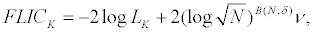(4)

where, LK is the log likelihood of the data evaluated at the posterior mean estimates for each function fi, i=1,…,I; N is the total number of observations defined in Equation (1). The number of free parameters in the model (ν) is the number of shape functions gk (note that we do not consider the number of parameters in the knot and spline coefficient vectors since we can use the posterior mean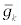from the sampler, post drift alignment, as our estimate for gk), plus the number of undetermined mixing proportions (K−1 free parameters), the total number of self-modeling coefficients in the 4×1 vector θi for all I subjects, and the common variance parameter σ2. The bivariate ratio function B(N,δ) and penalty statistic δ have been defined previously . For each MSIM considered, we can compute the fraction of between-component variability to within-component variability across the x values (or time points) and use these results to compute the average fraction of between-component variability to withincomponent variability (see formula in Supplementary Information).

#### Results

The MCMC implementation and post processing described in Sections 3-4 were executed for simulation studies and the motivating EPSPs example using R .

Simulations

We simulated data to identify how well the algorithm discriminated two separate, but similar functions. In each sample dataset we used the following underlying shape functions: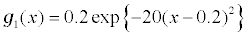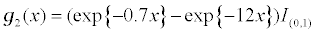These functions are similar to some parametric models used in the biology literature for EPSPs, and are quite similar in shape. Figure 3 shows the two underlying functions. The two functions are similar in shape, but they are clearly not identical. In other words, these two shape functions are clearly not self-modeling versions of each other. Although g1 is defined as a symmetric function, its alignment with g2 makes it appear asymmetric over the range of x. The function g2 is not differentiable at x=0 (note self-modeling versions with a slight shift are quite similar, so this cusp could appear at a different x value in the estimate, see the discussion of identifiability in Section 2.2), which creates a point where multiple knots are desirable. This is a situation where adaptive knot splines are more effective than using known knots.

Figure 3: Shape functions used for simulations. These two functions are aligned versions of g1(x)=0.2exp{−20(x−0.2)2} and g2(x)=(exp{−0.7x}– exp{−12x}) I(0,1).They are similar, but certainly NOT identical, in shape. Note also g2 is not differentiable at the initial rise, which creates a situation where multiple knots are desirable in that region. The simulations consisted of generating 40 datasets, each with 100 functions, 50 of which are selfmodeling versions of g1 with error and 50 of which are self-modeling versions of g2 with error.

Each sample dataset consisted of 100 functions, 50 generated from self-modeling versions of g1 and 50 from self-modeling versions of g2. The self-modeling coefficients (a,b,c,d) were randomly chosen for each function in each simulation to provide a range of overlapping functions. The range of values from which a,b,c,d were selected consisted of (3.0,7.0), (−0.15,0.10), (0.15,0.90), and (−0.06,0.80), respectively. The x values for each function correspond to 121 equally-spaced points in the interval (0,1). Forty simulated datasets of 100 functions were generated, 20 of which had an error standard deviation of σ=0.15 while the remaining 20 had an error standard deviation of σ=0.05. One sample dataset (for σ=0.05) is shown in Figure 4a. Figure 4b shows the same dataset color coded by the appropriate γ function. It is certainly not obvious from the data alone which γ is appropriate. The first- and second-stage MCMC algorithms from Equation (3) were run on each simulated dataset. The first-stage sampler was run for 80,000 iterations with the first 20,000 removed as burnin (this is perhaps excessive, but burn-in is long in this context and we were quite conservative). The second sampler was run for 30,000 iterations with the first 10,000 iterations removed. For each simulated dataset we computed the assessable quantities described in Section 4 (g1 and g2 estimated up to shape invariance, the individual functions fi, the probabilities of correct classification and error variance σ2).

Figure 4: A sample of 100 simulated functions. There are 50 self-modeling versions of each g1 and g2 mixed into the dataset shown in the left pane (a). The inferential problem is to separate the traces and simultaneously estimate the underlying shape functions. The right pane (b) shows the same dataset but colored according to the underlying groups (unknown in practice but here the red and blue functions are self-modeling versions of g1 and g2, respectively). The different groups are simulated so that they do not separate in groups based on height, width, or other functionals, but only by shape.

Figure 5 shows the estimated g1 and g2 plotted against the group assignments (for plotting, each function was assigned to the group that functions spent the most time assigned to in the second MCMC run) for the simulated dataset from Figure 4. The diagonal panes in Figure 5 show the functions (aligned) assigned to group 1 plotted against the fitted g1 and the functions assigned to group 2 (aligned) plotted against the fitted g2. The off-diagonal panes show each gk plotted against the functions which were NOT assigned to that group.

Figure 5: Results for simulated dataset. The four panels show the functions assigned to each group versus the fitted curves for g1 and g2. The diagonal panes (a) and (d) show the functions assigned to the corresponding g (e.g. the functions assigned to group 1 with g1 overlaid, and the functions assigned to group 2 with g2 overlaid). In contrast, the off-diagonal panes in (b) and (c) correspond to the functions overlaid with the "other" g (so the functions assigned to group 1 with g2 overlaid, and the functions assigned to group 2 with g1 overlaid).

Overall, correct classification rates for the functions were quite high and had similar rates across the simulations for both the first and second MCMC runs. Of the 20 datasets generated with lower noise σ=0.05, all but 3 had all 100 functions classified correctly. For those 3 datasets, 2 had 99 functions correctly classified and the other had 97 functions correctly classified. For the 20 datasets with σ=0.15, there was more variability because of the increased noise but there were still high correct classification rates, ranging from a low of 77 classified correctly to a high of 94.

An improvement in switching was defined as moving from the current state to the candidate state more often during post-burnin iterations in the second MCMC, compared to movement in the first MCMC. For higher noise (σ=0.15) data, all functions had improved acceptance rates. In lower noise (σ=0.05) data, switching improved in all but 3 datasets. For those 3 datasets, there were 4, 7, and 25 functions that did not improve their acceptance rates in the second run.

We calculated FLIC from Equation  for each simulation using one-group and two-group results. All lower-noise simulations favored including two distinct shape functions g1 and g2 over a single shape g. Eighteen of the higher-noise datasets favored the two-group model while 2 of the datasets had FLIC results favoring the single group model.

Classifying firings from synaptic transmission data

Of the 61 firings, 31 were classified to group 1 over 50% of the time while the other 30 were classified to group 2 over 50% of the time (to make sure the algorithm simply didn't split the 61 functions in half arbitrarily, we also fit subsets of the functions and found consistent assignments). Figure 6a is identical to Figure 1a except the functions are color coded into group 1 (red) and group 2 (blue). Acceptance probabilities were increased in the second MCMC run, but group membership changed for only 2 of the 61 firings from the first run to the second run.

Figure 6: Application to synaptic transmission data (a) The synaptic transmission data from Figure 1a, color coded into the groups identified by the Mixture of Self-Modeling regressions algorithm (a function was classified in a group if more than 50 % of the MCMC iterations placed it in that group). These groups do not appear to separate by peak amplitude, latency, or other commonly used functionals. (b) The synaptic transmission data from Figure 1b, aligned and color coded for the groups identified by the Mixture of Self-Modeling regressions model. The distinction between the two groups appears to be in the rate of increase in the rise and the rate of decrease in the descent. Functions in group 1 (red) have a faster rise and slower descent, while functions in group 2 (blue) have a slower rise and faster descent.

There is no obvious pattern to the assignments in Figure 6a (e.g. group 1 traces are not generally higher, wider, etc.). When we construct the plot analogous to the aligned data in Figure 1b, we do see a pattern emerge. In Figure 6b, the central difference in the groups appears near the aligned peaks. Group 1 (in red) shows a quick increase, followed by a slower decrease after the peak. In contrast, group 2 (in blue) has a slower increase to the peak, but a quicker decrease from the peak. Figure 7 shows the individual estimates of g1 and g2 plotted against the functions assigned (here meaning greater than 0.5 posterior probability) to each group. Thus the diagonal panels in this figure correspond to good fits (e.g. the functions assigned to group 1 with g1 overlaid, and the functions assigned to group 2 with g2 overlaid); the off diagonal panels show the differences between the groups (e.g. the function assigned to group 1 with g2 overlaid, and the functions assigned to group 2 with g1 overlaid). We compared the model results from the FLIC for a single shape versus two shapes and found the model with two shapes was more favorable. Again, the two different shapes are apparent in the graph.

Figure 7: Group assignments and fitted firings The four panels show the functions assigned to each group versus the fitted curves for g1 and g2 from the synaptic transmission data. The diagonal panes (a) and (d) show the functions assigned the corresponding g (e.g. the functions assigned to group 1 with g1 overlaid, and the functions assigned to group 2 with g2 overlaid). In contrast, the off-diagonal panes (b) and (c) correspond to the functions overlaid with the "other" g (so the functions assigned to group 1 with g2 overlaid, and the functions assigned to group 2 with g1 overlaid). This illustrates the differences in structure between g1 and g2.

#### Discussion

Fitting an MSIM allows for multiple shapes to be present in a sample of functions. In substantive contexts like the synaptic transmission data, finding these groups has a direct impact on questions like the number of active zones in a synapse. The algorithm proposed in this paper behaves well in simulations and provides results for the synaptic transmission data consistent with biological expectations.

Recent work by Zhang and Telesca  focuses on clustering and general registration of functional data where the number of knots and their locations are specified a priori and common to all underlying shapes. They show this approach works well for clustering growth data and gene expression curves. Our application to EPSPs and simulation studies address settings where the underlying shape functions are quite similar but perhaps one or more of the shape functions are not differentiable over the entire interval of interest. In these settings, we have found it advantageous to use BARS, compared to using known knots, as adaptive methods can include multiple knots to estimate cusps or other sharp changes in curvature.

The degree of switching (i.e. changes in acceptance rates) generally increased from the first MCMC to the second MCMC but appeared to depend on overall noise in the data. These results are consistent with existing literature on tuning proposal distributions . It is possible that other methods of "data-driven" tuning which incorporate overall variance in the MSIM may be necessary to further improve acceptance rates in the RJMCMC scheme .

Substantively, the finding of groups is expected, as such a finding can be directly linked to the underlying structural entities which are responsible for efficacy in chemical synaptic transmission in general [12,25,26]. However, if one fits a model with two groups, then two groups will appear in the results. While we were able to adapt an existing information criterion for this assessment  and obtain reasonable results, in the future it will be important to formally develop an inferential procedure for MSIMs. Fortunately for the synaptic transmission data, simpler mixture models have also detected two groups .

In univariate Gaussian mixture models, there are various model selection criteria that could be readily supplied [18,27] or one could use reversible-jump samplers to move between models with differing numbers of components in a manner similar to a previously-described approach . In our context it is unclear how to implement a model selection criteria that requires the number of parameters in the model, as the spline formulation includes the number of knots as one of the unknown parameters. In this work, we substitute these unknown parameters with the K parameters corresponding to estimatingfor k=1,…,K. Even simplistic criteria such as investigating the MSE between functions, or more precisely the use of χ2 or noncentral χ2 distributions, is complicated by the differing scaling produced by the self-modeling coefficients for each function. It would be of interest in future work to formally incorporate spline complexity in the fit statistic evaluation.

#### Acknowledgements

This research was supported by NCRR(NIH) Grant P20 RR16481. The authors are grateful to Richard Charnigo, PhD, for sharing implementation code for the flexible information criterion. The authors thank the reviewers for their comments, which have substantially improved the paper.

#### References

Select your language of interest to view the total content in your interested language

### Article Usage

• Total views: 11775
• [From(publication date):
specialissue-2015 - May 20, 2019]
• Breakdown by view type
• HTML page views : 7999
• PDF downloads : 3776

## Post your commentCan't read the image? click here to refresh
###### Peer Reviewed Journals

Make the best use of Scientific Research and information from our 700 + peer reviewed, Open Access Journals

International Conferences 2019-20

Meet Inspiring Speakers and Experts at our 3000+ Global Annual Meetings

Top